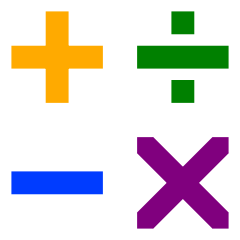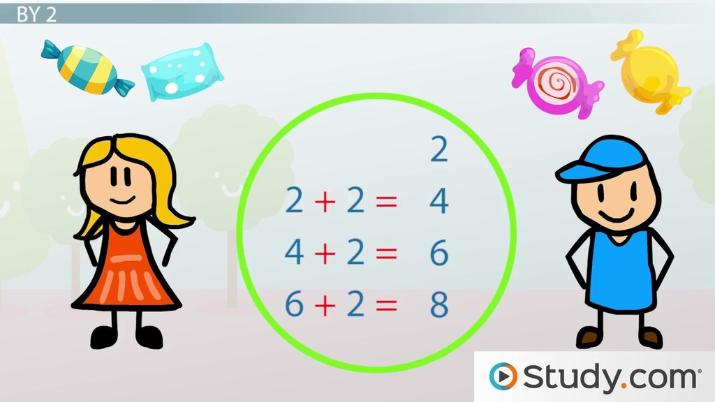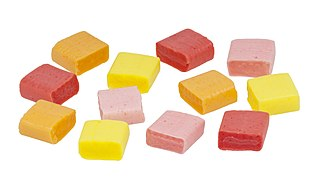# What is Divisibility in Math?

Elizabeth Coleman, Yuanxin (Amy) Yang Alcocer
• Author
Elizabeth Coleman

Elizabeth Coleman has a Bachelor's in Environmental Science and Policy from the University of South Florida. She has written education material for college geography courses and conducted scholarly research on natural disaster risk and management.

• Instructor
Yuanxin (Amy) Yang Alcocer

Amy has a master's degree in secondary education and has been teaching math for over 9 years. Amy has worked with students at all levels from those with special needs to those that are gifted.

In this lesson, learn what numbers are divisible by 2, 3, and 4. Understand how to know if a number is divisible by 3 or 4 and how to perform divisibility tests. Updated: 12/13/2021

Show

## Divisibility

The four basic functions of arithmetic, or the study of numbers, are addition, subtraction, multiplication, and division. This lesson will focus on division.In a division problem, there are three important numbers: the dividend, the divisor, and the quotient. The dividend is divided by the divisor to get the quotient. For example,

{eq}12 \div 3 = 4 {/eq}

In this problem, 12 is the dividend, 3 is the divisor, and 4 is the quotient. Twelve is divided by 3 to get 4; in other words, 12 is divisible by 3.

But what does it mean for a number to be divisible? A number is divisible by another number when it can be split equally into parts of that amount with no remainder. A remainder in division is a value left over when the dividend is not divisible by the divisor.

In the above example, 12 can be split into 4 equal parts of 3. This can be proven by adding 3 together 4 times: 3 + 3 + 3 + 3 = 12.

## Divisibility

What is divisibility? Divisibility is being able to be divided equally by a certain number. Think of sharing a bunch of candies that you have with your friends. If you have 3 friends and 3 candies, then you can give each friend 1 candy. Everyone gets an equal share. In math, we call this divisibility by 3. If you can't give your friends an equal share, then we say we don't have divisibility by 3.

We also use the word 'divisible.' We will usually say that something is divisible by something else. So, we will say that 3 is divisible by 3 because you can divide 3 candies by 3 friends equally. Remember what the symbol looks like for division? Yes, it is a horizontal line with a dot on top and a dot on the bottom. In this video lesson, we will look at what numbers can be divided by 2, 3, and 4. Are you ready to begin?An error occurred trying to load this video.

Try refreshing the page, or contact customer support.

Coming up next: Divisibility by 5, 6, and 7

### You're on a roll. Keep up the good work!

Replay
Your next lesson will play in 10 seconds
• 0:03 Divisibility
• 1:04 By 2
• 2:36 By 3
• 3:33 By 4
• 4:06 Lesson Summary
Save Save

Want to watch this again later?

Timeline
Autoplay
Autoplay
Speed Speed## Numbers Divisible by 2

When numbers are divisible by 2, they can be divided equally by 2 with no remainder. Luckily, there is a divisibility test that can be performed to check whether a number is divisible by 2, no matter how small or large it is. If the number passes the test, it can be divided by 2!

According to the divisibility test, all even numbers are divisible by 2. Even numbers end in 0, 2, 4, 6, or 8. This means that 0, 2, 4, 6, and 8 are all divisible by 2. Additionally, any number that ends in 0, 2, 4, 6, or 8 is also divisible by 2.

Here are some examples:

#### Use the divisibility test to determine if 4,823 is divisible by 2.

The number 4,823 ends in 3, which is not an even number. Therefore, 4,823 is not divisible by 2.

#### Use the divisibility test to determine if 106 is divisible by 2.

The number 106 ends in 6, which is an even number. Therefore, 106 is divisible by 2.

## Numbers Divisible by 3

Numbers that are divisible by 3 can be split into equal parts of 3 with no remainder, like 12 in the earlier example. Other numbers divisible by 3 include 9, 15, 18, 21, and so on.

### How to Know if a Number is Divisible by 3

Just like with 2, there is a divisibility test to determine whether a number is divisible by 3. This test is a bit more complicated than the test for divisibility by 2, though.

To find whether a number is divisible by 3, first add all the digits of the number. Digits are the individual numbers from 0 to 9 that make up the larger number.

Once the sum is found, check to see if the sum is divisible by 3. If the sum is divisible by 3, the original number will be, too. If the sum is not divisible by 3, the original number won't be, either.

To test this rule, here are some examples:

#### Use the divisibility test to determine whether 90,354 is divisible by 3.

First, add the digits of the number 90,354 together. 9 + 0 + 3 + 5 + 4 = 21.

21 is divisible by 3. Therefore, 90,354 is also divisible by 3.

#### Use the divisibility test to determine whether 104,789,332 is divisible by 3.

First, add the digits of the number 104,789,332 together. 1 + 0 + 4 + 7 + 8 + 9 + 3 + 3 + 2 = 37.

## By 2

Let's stick to our sharing candies with friends example. In this case, divisibility by 2; we have 2 friends that we want to share candies with. Can you think of a number of candies that can be divided equally between these two friends? Is it 2? Yes, if you have 2 candies, you can definitely share the 2 candies equally between your 2 friends.

How many candies would each friend get? 1. So, 2 is divisible by 2, and 2 divided by 2 equals 1 since that is the number of candies that each friend got. Think of splitting the number of candies you have evenly into 2 groups. If you can do that, then the number is divisible by 2.

What's another number that is divisible by 2? Let's try 4. How can you divide four candies equally between 2 friends? Each friend can get 2 candies. So, we have 4 divided by 2 is 2. What other numbers have divisibility by 2? 6, 8, 10, 12, and so on. Do you see a pattern? Yes, each number is the previous number plus 2. You can continue this pattern to find even more numbers that are divisible by 2.

## By 3

Let's continue on to the number 3. Now you have 3 friends. How many candies do you need so that you can share them equally between your friends? The first number is 3, since this means that each friend will get 1 candy each. So, 3 is divisible by 3. 3 divided by 3 is 1.

To unlock this lesson you must be a Study.com Member.

Video Transcript

## Divisibility

What is divisibility? Divisibility is being able to be divided equally by a certain number. Think of sharing a bunch of candies that you have with your friends. If you have 3 friends and 3 candies, then you can give each friend 1 candy. Everyone gets an equal share. In math, we call this divisibility by 3. If you can't give your friends an equal share, then we say we don't have divisibility by 3.

We also use the word 'divisible.' We will usually say that something is divisible by something else. So, we will say that 3 is divisible by 3 because you can divide 3 candies by 3 friends equally. Remember what the symbol looks like for division? Yes, it is a horizontal line with a dot on top and a dot on the bottom. In this video lesson, we will look at what numbers can be divided by 2, 3, and 4. Are you ready to begin?

## By 2

Let's stick to our sharing candies with friends example. In this case, divisibility by 2; we have 2 friends that we want to share candies with. Can you think of a number of candies that can be divided equally between these two friends? Is it 2? Yes, if you have 2 candies, you can definitely share the 2 candies equally between your 2 friends.

How many candies would each friend get? 1. So, 2 is divisible by 2, and 2 divided by 2 equals 1 since that is the number of candies that each friend got. Think of splitting the number of candies you have evenly into 2 groups. If you can do that, then the number is divisible by 2.

What's another number that is divisible by 2? Let's try 4. How can you divide four candies equally between 2 friends? Each friend can get 2 candies. So, we have 4 divided by 2 is 2. What other numbers have divisibility by 2? 6, 8, 10, 12, and so on. Do you see a pattern? Yes, each number is the previous number plus 2. You can continue this pattern to find even more numbers that are divisible by 2.

## By 3

Let's continue on to the number 3. Now you have 3 friends. How many candies do you need so that you can share them equally between your friends? The first number is 3, since this means that each friend will get 1 candy each. So, 3 is divisible by 3. 3 divided by 3 is 1.

To unlock this lesson you must be a Study.com Member.

#### What is it called when a number is divisible by 2?

When a number is divisible by 2, it is an even number. Even numbers include 0, 2, 4, 6, and 8, along with any larger number that ends in 0, 2, 4, 6, or 8.

#### Are all numbers that end in 4 divisible by 4?

Not all numbers that end in 4 are divisible by 4. Examples of numbers ending in 4 that cannot be divided equally by 4 are 14, 34, and 54.

### Register to view this lesson

Are you a student or a teacher?

Back

### Resources created by teachers for teachers

Over 30,000 video lessons & teaching resources‐all in one place.Video lessonsQuizzes & WorksheetsClassroom IntegrationLesson Plans

I would definitely recommend Study.com to my colleagues. It’s like a teacher waved a magic wand and did the work for me. I feel like it’s a lifeline.

Jennifer B.
TeacherCreate an account to start this course today
Used by over 30 million students worldwide